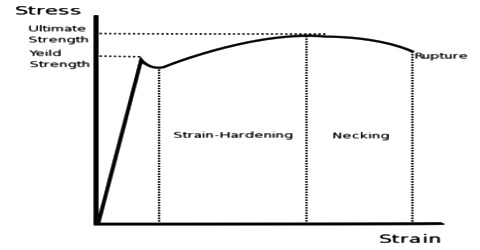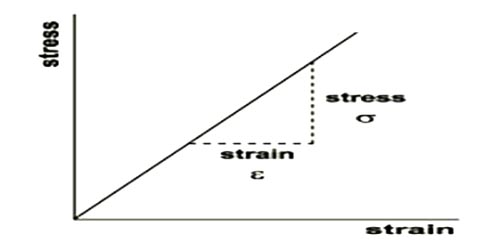# Hooke’s Law of Stress and Strain

Hooke’s Law

Famous scientist Robert Hooke observed close relation between stress and strains. Is 1678 he expressed this relation by a law. It is called Hooke’s law. This Law is a principle of physics that states that the force needed to extend or compress a spring by some distance is proportional to that distance. The law is stated below:

“Within the elastic limit, the stress applied is proportional to the strain produced”. On the application of stress on an elastic body, the strain experienced by the body (amount of deformation) is directly proportional to the stress applied.

Mathematically it can be written as; stress strain

or, stress = constant x strain

or, stress / strain = constant

This constant is called co-efficient of elasticity. It’s also called elastic constant.Explanation: When force is applied on a body, then there appears strain or deformation. If force does not exceed the elastic limit, according to Hook’s law, more the strain of a body, more is the stress. From the definition of stress we know force per unit area is called stress. So, more the applied force per unit area, more will be strain; that means, its length, volume and size will change more. The amount of strain will be same as the amount of force applied on a unit area.

This law had many important practical applications, with one being the creation of a balance wheel, which made possible the creation of the mechanical clock, the portable timepiece, the spring scale and the manometer. Also, because it is a close approximation of all solid bodies, numerous branches of science and engineering as also indebted to Hooke for coming up with this law. These include the disciplines of seismology, molecular mechanics and acoustics.